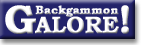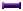TheoryForum Archive : Theory

 Janowski's formulas

 From: Joern Thyssen Address: jth@chem.ou.dk Date: 2 August 2000 Subject: Janowski's formulas for cubeful equity Forum: rec.games.backgammon Google: 39881471.6E3AB38B@chem.ou.dk

```Hi

I've got hold of three issues for Hoosier BG Club magazine from
1993-1994 (Volumn X, no. 6  (1993); Volumn XI, no. 1 (1994); Volume XI,
no. 2 (1994)) where Rick Janowski writes about take points in money
game.

I've tried to reproduce Rick Janowski's formulas but I have some
trouble.

W is the average points won pr. game, L is the average loss pr. game.
All the formulas are for money game without the Jacoby rule [Janowski
does give formulas for play with Jacoby w/o beavers, but let's not
complicate things more than necesary].

Take points:

Eq 1: dead cube take point: TP = (L-0.5)/(W+L)
Eq 2: live cube take point: TP = (L-0.5)/(W+L+0.5)
Eq 3: general take point: TP = (L-0.5)/(W+L+0.5x), where 0<=x<=1
(x=0 gives dead cube model, x=1 gives live cube model).

Cubeless take equity:

Eq 4: E_{take} = TP ( W + L ) - L

Equation 4 is just a special case of the cubeless equity:

E = p W -  ( 1 - p ) L
= p ( W + L ) - L

with p = TP, you get Eq. 4.

Cubeful equity:

Eq 5: E_O = E_{I own cube} = Cv [ p (W+L+0.5x) - L]
Eq 6: E_{Opp own cube} = Cv [ p (W+L+0.5x) - L - 0.5x ]
Eq 7: E_{cent. cube} = 4 Cv / (4-x) [p (W+L+0.5x) - L - 0.25]

(Cv is the current value of the cube, p is the probability that you'll
win this game).

But Eqs. 5-7 is giving me trouble. Well, so far only Eq. 5, because I
didn't want to check the others before I could reproduce Eq. 5 [
Actually, Eq.
6 is easily derived from Eq. 5 (just interchance W <-> L, p <-> 1-p, and
voila!].

I would derive Eq. 5 as follows:

At p = 0: my cubeful equity is -L,
at p = TP: my cubeful equity is +1,
and do a linear interpolation inbetween.

Then I arrive at:

E_O = C_V [ p ( 1 + L ) / ( L + 0.5 + 0.5 x ) ( W + L + 0.5 x ) - L ]

whereas Janowski gets

Eq. 5: E_O = C_V [ p ( W + L + 0.5x ) - L ]

If I plug in p = 0 in Janowski's formula E_O = -L. Fine!

But with p = TP = 1 - (W-0.5)/(W+L+0.5x) (I'm using my opponents
takepoint, since I own the cube) I get:

E_O = 0.5x + 0.5 (Janowski) != 1 (for x != 1 ).
E_O = 1          ("my" formula).

So, does any of you know how Janowski derives his Eq. 5?
I appreciate any help.

Joern Thyssen
```

 Phill Skelton  writes: ```Part of the problem is that your cube efficiency and your opponents may well not be the same, or even close to each other. Sticking x = 0 into the equations evidently implies that you can't double at all as it reproduces the cubeless equity. OTOH if you are able to double, your opponent has a dead cube and p = 0.75 (i.e. you are at his dead cube take-point, when W = L = 1) then your equity is clearly 1, and you are offering a perfectly efficient double. The assumptions Jankowski uses evidently means that the formula is not applicable to this situation. OTOH the approach taken by Joern makes a different assumption (that the player holding the cube has a perfectly efficient double and that the parameter x only applies to your opponent). In the real world x is different for each player and varies depending on the situation - you might try to model it as a function of the volatility and the distance from the ideal doubling point, but that begins to introduce even more variables into the equations. I think that the bottom line is that it's just not possible to come up with a formula for cubeful equities that is generally applicable and sufficiently simple to be useful, but perhaps the 'special case' equities may be good enough for many situations. Phill ```

 �ystein Johansen  writes: ```Phill Skelton wrote: > In the real world x is different for each player and varies depending on > the situation - you might try to model it as a function of the volatility > and the distance from the ideal doubling point, but that begins to > introduce even more variables into the equations. This is the real interesting part. Joern, does your article say anything about this? I have done some small experimets to calculate a good value for x, as function off the position. This following values are based on Jellyfish evaluations and conversations with a strong player. Pure race: x ~ 0.65 Holding games: x ~ 0.5 Deep backgames: x ~ 0.4 Faceing an attack: x ~ 0.9 Last roll positions: x = 0.0 (obviously) It is hard to estimate a good value for x in late bearoff positions. I think maybe it should be increasing with the expected number of rolls left for the accepting/droping player. Take a "~one-roll-each" position. Then if this player expects to get off in 1.25 rolls, (27 good rolls - 9 bad rolls), the value of x should be 1.0. Of course you can try to make this values more sophisticated. They're far for perfect. Best regards, �ystein Johansen ```

 Joern Thyssen  writes: ```> Joern, does the article say anything about this? I quote: "Finding accurate values for x is a difficult, almost impossible task. However, we can make estimates of /typical/ values for /typical/ situations. In my opinion, for the majority of /typical/ positions, x will commonly be between 1/2 and 3/4, with 2/3 being a /normal/ value." > Joern could you define more clearly the W and L? Are they the > possibilities for you winning and losing the game? I 'm afraid I don't > understand that "W is the average points won pr. game, L is the average > loss pr. game". An arithmetic example would be of great help. W = p(win) + p(win gammon/backgammon) + p(win backgammon) ----------------------------------------------------- p(win) Analogous for L. For example, in a pure race (with no contact): W = p(win) = 1 ------ p(win) Suppose a evaluation gives: win 50% g/bg 11% bg 0% (a typical opening situation) W = 50 + 11 = 1.22 ------- 50 ```

 Joern Thyssen  writes: ```> Cubeful equity: > > Eq 5: E_O = E_{I own cube} = Cv [ p (W+L+0.5x) - L] > Eq 6: E_{Opp own cube} = Cv [ p (W+L+0.5x) - L - 0.5x ] > Eq 7: E_{cent. cube} = 4 Cv / (4-x) [p (W+L+0.5x) - L - 0.25] > > (Cv is the current value of the cube, p is the probability that you'll > win this game). After personal communication with Rick Janowski I now know how to derive these formulae: E = (1-x) (E_dead) + x (E_live) where E_dead = p * (W+L) - L (equal to the cubeless equity) E_live = p * (W+L+0.5) - L (by doing linear interpolation between (p=0,E=-L) and (p=opponent take point=1-(W-0.5)/(W+L+0.5)=(L+1)/(W+L+0.5), E=+1). This gives E_O = (1-x) ( p (W+L) - L ) + x ( p (W+L+0.5) - L ) = p (W+L+0.5x) - L Joern ```

### Theory

Derivation of drop points  (Michael J. Zehr, Apr 1998)
Double/take/drop rates  (Gary Wong, June 1999)
Drop rate on initial doubles  (Gary Wong, July 1998)
Error rate--Why count forced moves?  (Ian Shaw+, Apr 2009)
Error rates--Repeated ND errors  (Joe Russell+, July 2009)
Inconsistencies in how EMG equity is calculated  (Jeremy Bagai, Nov 2007)Janowski's formulas  (Joern Thyssen+, Aug 2000)
Janowski's formulas  (Stig Eide, Sept 1999)
Jump Model for money game cube decisions  (Mark Higgins+, Mar 2012)
Number of distinct positions  (Walter Trice, June 1997)
Number of no-contact positions  (Darse Billings+, Mar 2004)
Optimal strategy?  (Gary Wong, July 1998)
Proof that backgammon terminates  (Robert Koca+, May 1994)Solvability of backgammon  (Gary Wong, June 1998)
Undefined equity  (Paul Tanenbaum+, Aug 1997)Under-doubling dice  (Bill Taylor, Dec 1997)Variance reduction  (Oliver Riordan, July 2003)From GammOnLineLong messageRecommended readingRecent addition

 Book Suggestions Books Cheating Chouettes Computer Dice Cube Handling Cube Handling in Races Equipment Etiquette Extreme Gammon Fun and frustration GNU Backgammon History Jellyfish Learning Luck versus Skill Magazines & E-zines Match Archives Match Equities Match Play Match Play at 2-away/2-away Miscellaneous Opening Rolls Pip Counting Play Sites Probability and Statistics Programming Propositions Puzzles Ratings Rollouts Rules Rulings Snowie Software Source Code Strategy--Backgames Strategy--Bearing Off Strategy--Checker play Terminology Theory Tournaments Uncategorized Variations